Math resources Geometry Area

Area of a parallelogram

# Area of a parallelogram

Here you will learn about finding the area of a parallelogram, including compound area questions, questions with missing side lengths, and questions involving unit conversion.

Students will first learn about area of a parallelogram as part of geometry in 6th grade.

## What is the area of a parallelogram?

The area of a parallelogram is the amount of space inside the parallelogram. It is measured in units squared, or square units. ( cm^2, m^2, mm^2, etc.) It is calculated by multiplying the base of a parallelogram by the perpendicular height.

In order to find the area, you need to use the area formula:

\begin{gathered} \text { Area }=\text { base } \times \text { perpendicular height } \\\\ \text { Area }=b \times h \end{gathered}

For example,

\begin{aligned} \text { Area } & =\text { base } \times \text { perpendicular height } \\\\ & =8 \times 6 \\\\ & =48 \mathrm{~m}^2 \end{aligned}

A parallelogram is a quadrilateral ( 4 sided shape) with 2 pairs of parallel lines.

Opposite sides of a parallelogram are parallel. The parallel sides and opposite angles are congruent. The interior angles of the parallelogram add up to 360^{\circ}.

To understand the formula, you cut off the triangle on one end of the parallelogram and attach it to the other end to form a rectangle. The formula for the area of a rectangle is base multiplied by height.

Your final answer must be in square units. For example, square centimeters (cm^2), square meters (m^2), square feet (ft^2) etc.

### What is the area of a parallelogram?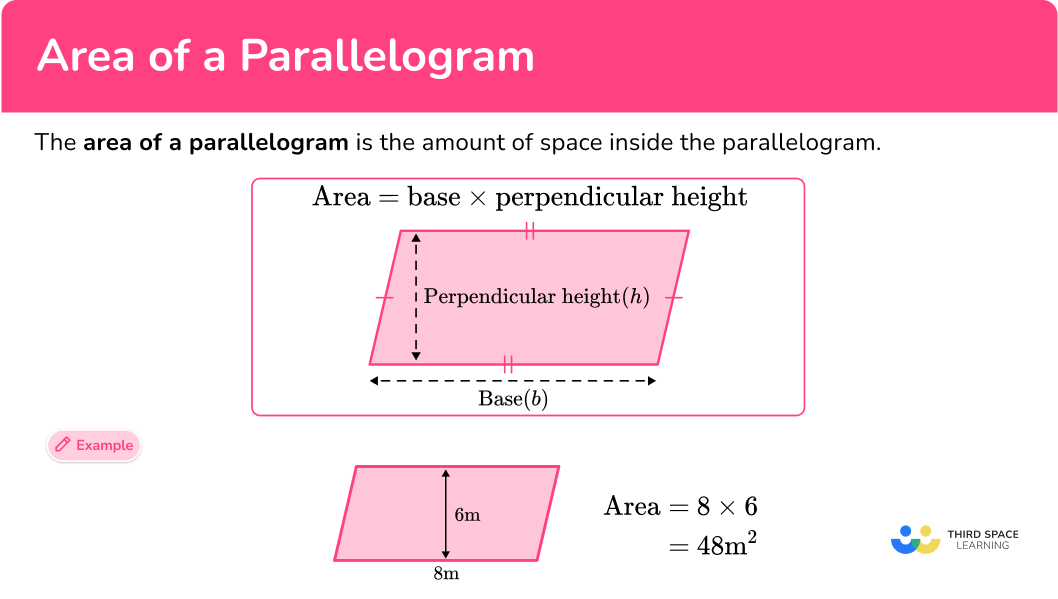## Common Core State Standards

How does this relate to 6th grade math?

• Grade 6 – Geometry (6.G.1)
Find the area of right triangles, other triangles, special quadrilaterals, and polygons by composing into rectangles or decomposing into triangles and other shapes; apply these techniques in the context of solving real-world and mathematical problems.

## How to find the area of a parallelogram

In order to find the area of a parallelogram by decomposing the shape:

1. Decompose the parallelogram into two triangles and a rectangle.
2. Move one triangle to the opposite side of the parallelogram so that the shape is now a rectangle.
3. Identify the base and height of the rectangle.
4. Write down the formula for the area of a rectangle.
5. Substitute the given values and calculate.

In order to find the area of a parallelogram using the formula:

1. Identify the base and the perpendicular height of the parallelogram.
2. Write down the formula for the area of a parallelogram.
3. Substitute the given values and calculate.

## Area of a parallelogram examples

### Example 1: decompose the parallelogram

Decompose the parallelogram and rearrange its part so that they form a rectangle. Then find the area.

1. Decompose the parallelogram into two triangles and a rectangle.

2Move one triangle to the opposite side of the parallelogram so that the shape is now a rectangle.

3Identify the base and height of the rectangle.

Base = 10 \, m

Height = 6 \, m

4Write down the formula for the area of a rectangle.

\text { Area }=\text { Base } \times \text { Height }

5Substitute the given values and calculate.

\begin{aligned} \text { Area }&=\text { Base } \times \text { Height } \\\\ &=10 \times 6 \\\\ &=60 \end{aligned}

\text { Area }=60 \mathrm{~m}^{2}

### Example 2: decompose the parallelogram

Find the area of the parallelogram below:

Decompose the parallelogram into two triangles and a rectangle.

Move one triangle to the opposite side of the parallelogram so that the shape is now a rectangle.

Identify the base and height of the rectangle.

Write down the formula for the area of a rectangle.

Substitute the given values and calculate.

### Example 3: decompose to find the area of a parallelogram requiring converting units

Decompose the parallelogram into two triangles and a rectangle.

Move one triangle to the opposite side of the parallelogram so that the shape is now a rectangle.

Identify the base and height of the rectangle.

Write down the formula for the area of a rectangle.

Substitute the given values and calculate.

### Example 4: find the area of a parallelogram using the formula

Identify the base and the perpendicular height of the parallelogram.

Write down the formula for the area of a parallelogram.

Substitute the given values and calculate.

### Example 5: use the formula to find the area of a parallelogram requiring converting units

Identify the base and the perpendicular height of the parallelogram.

Write down the formula for the area of a parallelogram.

Substitute the given values and calculate.

### Example 6: calculating base length given the area

Find the base length of the parallelogram below:

Identify the base and the perpendicular height of the parallelogram.

Write down the formula for the area of a parallelogram.

Substitute the given values and calculate.

### Example 7: word problem

A painter needs to paint a logo on the side of an office firm. The logo is composed of four identical parallelograms, as shown below. Each can of paint costs \\$1.20 and covers an area of 2.5m^2. How much will it cost to paint the logo?

Identify the base and the perpendicular height of the parallelogram.

Write down the formula for the area of a parallelogram.

Substitute the given values and calculate.

### Teaching tips for area of a parallelogram

• Provide students with a variety of problems, including word problems with a real-world context. Label parallelograms with the perpendicular height as well as the diagonal length, so that they need to correctly differentiate between the two in order to solve.

• Encourage students to follow the step-by-step process used above for each strategy. Have students write these steps in their math notebooks, or post them in the classroom for students to refer to.

### Easy mistakes to make

• Using incorrect units for the answer
A common error is to forget to include square units when asked to find area.

• Forgetting to convert measures to a common unit
Before using the formula for calculating the area of a parallelogram, students must look at the units given in the question. If different units are given, for example, length = 4 \, m and width = 3 \, cm, students must convert them both to cm or both to m.

• Using length of the diagonal when calculating area and not the perpendicular height of a parallelogram
Sometimes in a question, additional measurements are given that are not needed in order to find the area. Sometimes the length of one of the diagonal sides of the parallelogram will be given, which must be ignored. Only the base and perpendicular height measurements are needed to find the area.

### Practice area of a parallelogram questions

1. Find the area of the parallelogram below: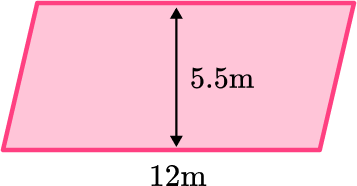17.5 \, m17.5 \, m^266 \, m^266 \, mMultiply the b \times h, which is 12 \times 5.5, which equals 66.

Since you are calculating area, the answer must be in square units.

2. Find the area of the parallelogram below: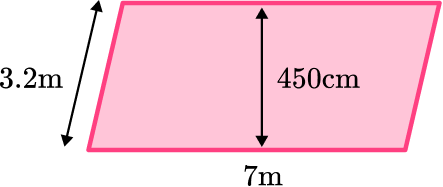3150 \, m^236.4 \, m^214.4 \, m^231.5 \, m^2Convert 450 \, cm into meters by dividing by 100.

Multiply the base length (7 \, m) by the converted perpendicular height (4.5 \, m) to equal 31.5 \, m^2.

Be careful not to multiply by the length of the diagonal instead of the perpendicular height.

3. The diagram represents a garden with a square fountain in the middle. Calculate the area of the shaded region of the garden.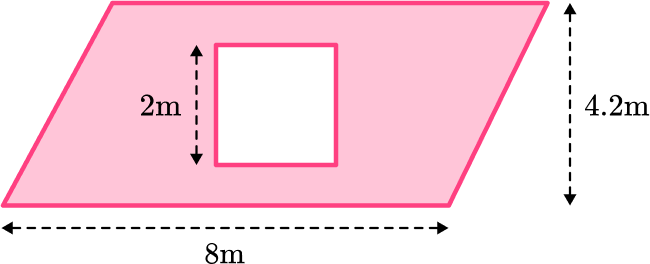29.6 \, m^233.6 \, m^212.2 \, m^210.2 \, m^2Find the area of the parallelogram by multiplying the base length times the height.

Find the area of the square by multiplying the base times the height.

Subtract the area of the square by the area of the parallelogram.

4. Calculate the length of the base of the parallelogram below: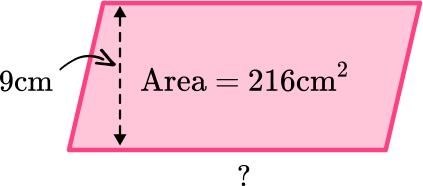1994 \, cm^224 \, cm24 \, cm^21994 \, cmDivide the area, 216 \, cm^2, by the perpendicular height of 9 \, cm.

Remember that you are not calculating the area so your final answer should not be in square units.

5. Calculate the area of the shaded region below: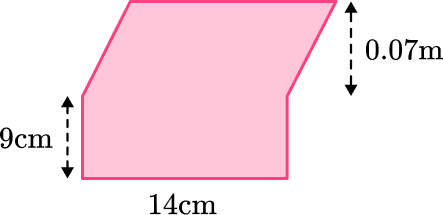126 \, cm^298 \, cm^2126.98 \, cm^2224 \, cm^2Convert all measures to cm.

Find the area of the parallelogram first by multiplying the base times the height.

Add on the area of the rectangle which is calculated by multiplying the length and the width.

6. A landscaper is planning a rectangular garden for a client. The garden will feature a patio and sandpit both in the shape of parallelograms. The remainder of the garden will be covered in grass. Below is a blueprint of the garden. What area of the garden will be covered in grass?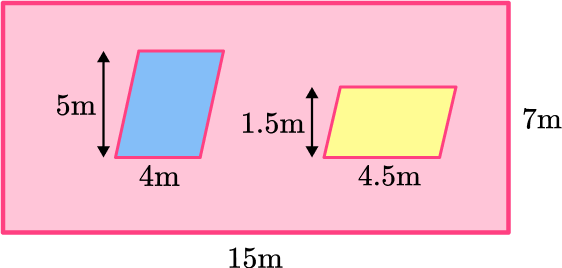105 \, m^278.25 \, m^285 \, m^2131.75 \, m^2First, find the area of the entire garden by multiplying its length by its width (15 \times 7 = 105).

Then, find the areas of the patio and the sandpit by multiplying base times perpendicular height for each one.

(5 \times 4 = 20, and 1.5 \times 4.5 = 6.75)

Subtract 20 and 6.75 from the total area, 105.

105-20-6.75 = 78.25m^2.

## Area of a parallelogram FAQs

How do you calculate the area of a parallelogram?

The area of a parallelogram is calculated by multiplying the base of a parallelogram times its perpendicular height.

What is the area of a parallelogram formula?

The formula for area of a parallelogram is
\text{ area } = \text{ base } \times \text{ perpendicular height. }

Can the adjacent sides of a parallelogram be right angles?

Yes, if the adjacent sides are right angles, this means the opposite sides are parallel, making the shape a parallelogram or more specifically a rectangle.

## Still stuck?

At Third Space Learning, we specialize in helping teachers and school leaders to provide personalized math support for more of their students through high-quality, online one-on-one math tutoring delivered by subject experts.

Each week, our tutors support thousands of students who are at risk of not meeting their grade-level expectations, and help accelerate their progress and boost their confidence.